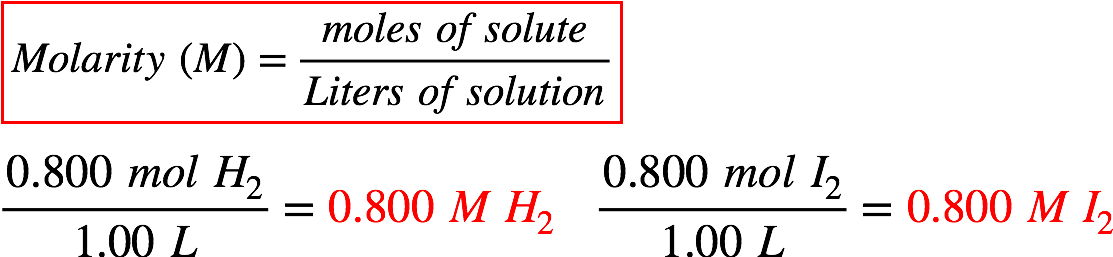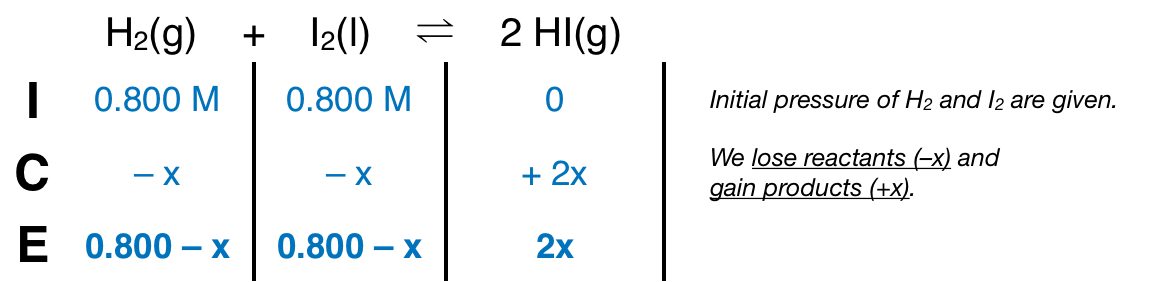# Problem: At a certain temperature, the equilibrium constant K c for this reaction is 53.3.H2 + I 2 → 2 HIAt this temperature, 0.800 mol of H2 and 0.800 mol of I2 were placed in 1.00L container to react. What concentration of HI is present at equilibrium?

###### FREE Expert Solution
96% (366 ratings)
###### FREE Expert Solution

We’re given the following equilibrium reaction:

H2 + I2(g)  2 HI; Kc = 53.3

We know that the initial amount of H2 and I2 is 0.800 mol. In a 1.00 L container, the concentration of each is:From this, we can construct an ICE table:The Kc expression for the reaction is:96% (366 ratings)###### Problem Details

At a certain temperature, the equilibrium constant K c for this reaction is 53.3.

H2 + I 2 → 2 HI

At this temperature, 0.800 mol of H2 and 0.800 mol of I2 were placed in 1.00L container to react. What concentration of HI is present at equilibrium?

What scientific concept do you need to know in order to solve this problem?

Our tutors have indicated that to solve this problem you will need to apply the ICE Chart concept. You can view video lessons to learn ICE Chart. Or if you need more ICE Chart practice, you can also practice ICE Chart practice problems.

What is the difficulty of this problem?

Our tutors rated the difficulty ofAt a certain temperature, the equilibrium constant K c for t...as medium difficulty.

How long does this problem take to solve?

Our expert Chemistry tutor, Jules took 4 minutes and 59 seconds to solve this problem. You can follow their steps in the video explanation above.

What professor is this problem relevant for?

Based on our data, we think this problem is relevant for Professor Kobko-Litskevitch's class at HUNTER.